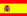You are here : > Home> Utilities > Mathematics & Statistics > Straight line depreciation method >

### Straight Line Depreciation Method

To calculate the monthly depreciation of an asset, by the straight line basis, enter the data: Asset historical cost or initial cost of depreciable asset, salvage value of the asset and the annual depreciation rate, in accordance with legal regulations.
Use the point as decimal separator. Example, for the number 35,132.42 enter 35132.42; The results are automatically displayed after you click on "Calculate"

 Straight Line Depreciation Method Asset historical cost: Salvage value of the asset: Year depreciation rate: Monthly depreciation: Annual depreciation: Period in months: